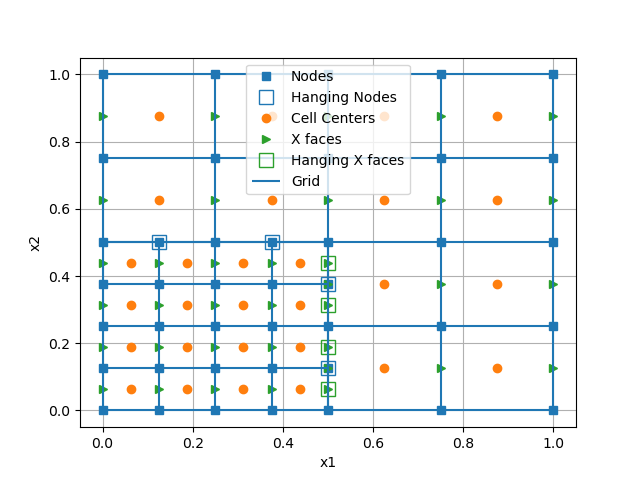You can give the refine method a function, which is evaluated on every cell of the TreeMesh.

Occasionally it is useful to initially refine to a constant level (e.g. 3 in this 32x32 mesh). This means the function is first evaluated on an 8x8 mesh (2^3).```import discretize
import matplotlib.pyplot as plt

def run(plotIt=True):
M = discretize.TreeMesh([8, 8])

def refine(cell):
xyz = cell.center
dist = ((xyz - [0.25, 0.25]) ** 2).sum() ** 0.5
if dist < 0.25:
return 3
return 2

M.refine(refine)
if plotIt:
M.plot_grid(nodes=True, centers=True, faces_x=True)
plt.legend(
(
"Nodes",
"Hanging Nodes",
"Cell Centers",
"X faces",
"Hanging X faces",
"Grid",
)
)

if __name__ == "__main__":
run()
plt.show()
```

Total running time of the script: ( 0 minutes 0.100 seconds)

Gallery generated by Sphinx-Gallery